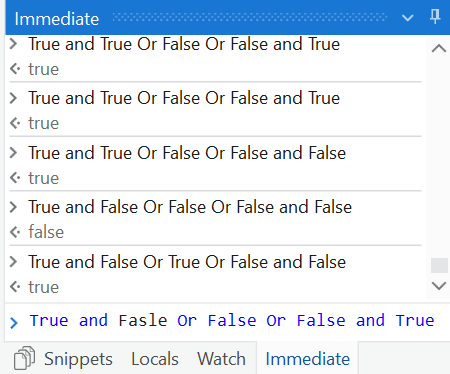# Multiple and ,or condition how to evaluate

Hi guys
Need your help to understand very basic question I am getting confuse with “and” “or” logic for example how to evaluate multiple " and or "if I have given condition

X = Y and Y= Z or a=b or c=d and d=e

What is outcome of give example i am also attaching my uipath logic can you please explain what is performing here ?

Hello,
Your condition is here divided in 3:
First sentence : row(“IsActive”).ToString.Trim.ToUpper.Equals(“YES”)
Second Sentence : row(“ProcessingMonth”).ToString.Trim.Equals(“EveryMonth”) or row(“ProcessingMonth”).ToString.Trim.contains(System.DateTime.Now.ToString(“MMM”))
Third sentence : row(“LastProceessedMonth”).ToString <> System.DateTime.Now.ToString(“MMMM”)

For your condition to be true you need all of these three sentences to be true
Your your second sentence to be true you need only one of the two conditions inside to be true

To use let me give you an example here

X = Y and Y= Z or a=b or c=d and d=e
Say True and True Or False Or False and True
then output is True
Say True and Fasle Or False Or False and True
then output is False

The middle 3 Y= Z or a=b or c=d are considered for OR and if atleast one is True the output will be true
Now if you want to segregate